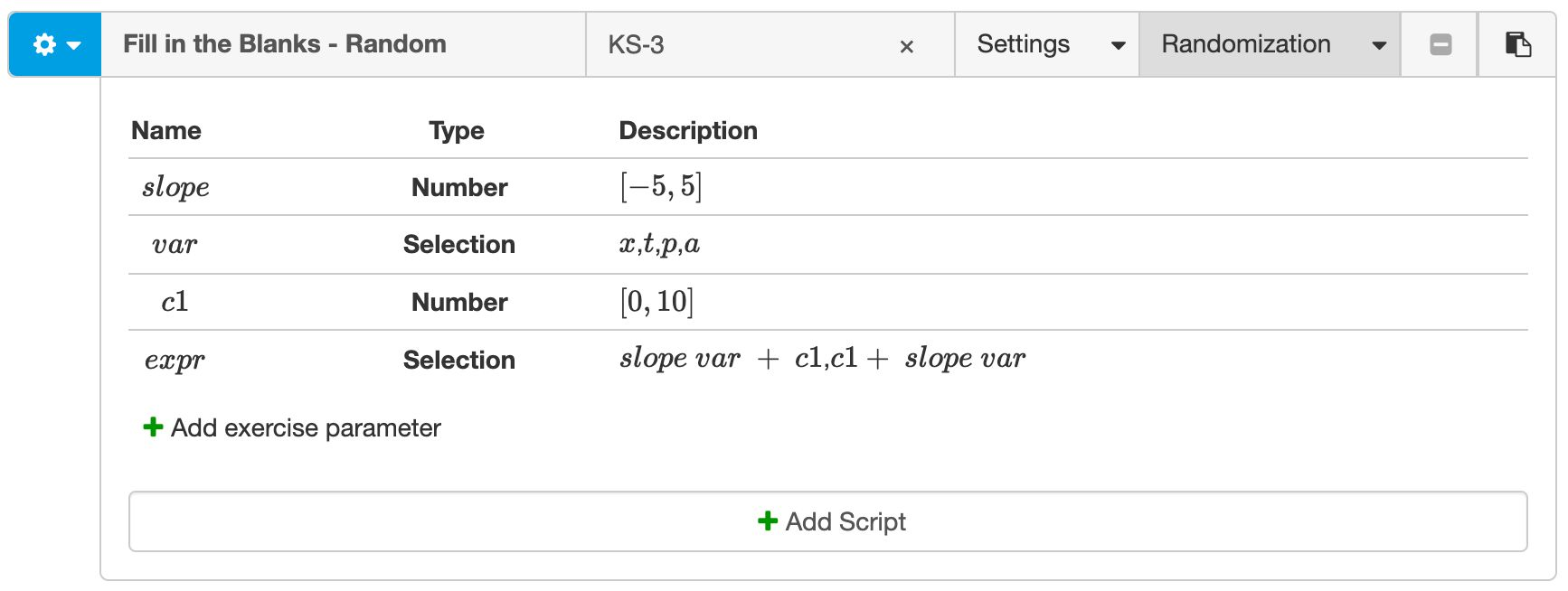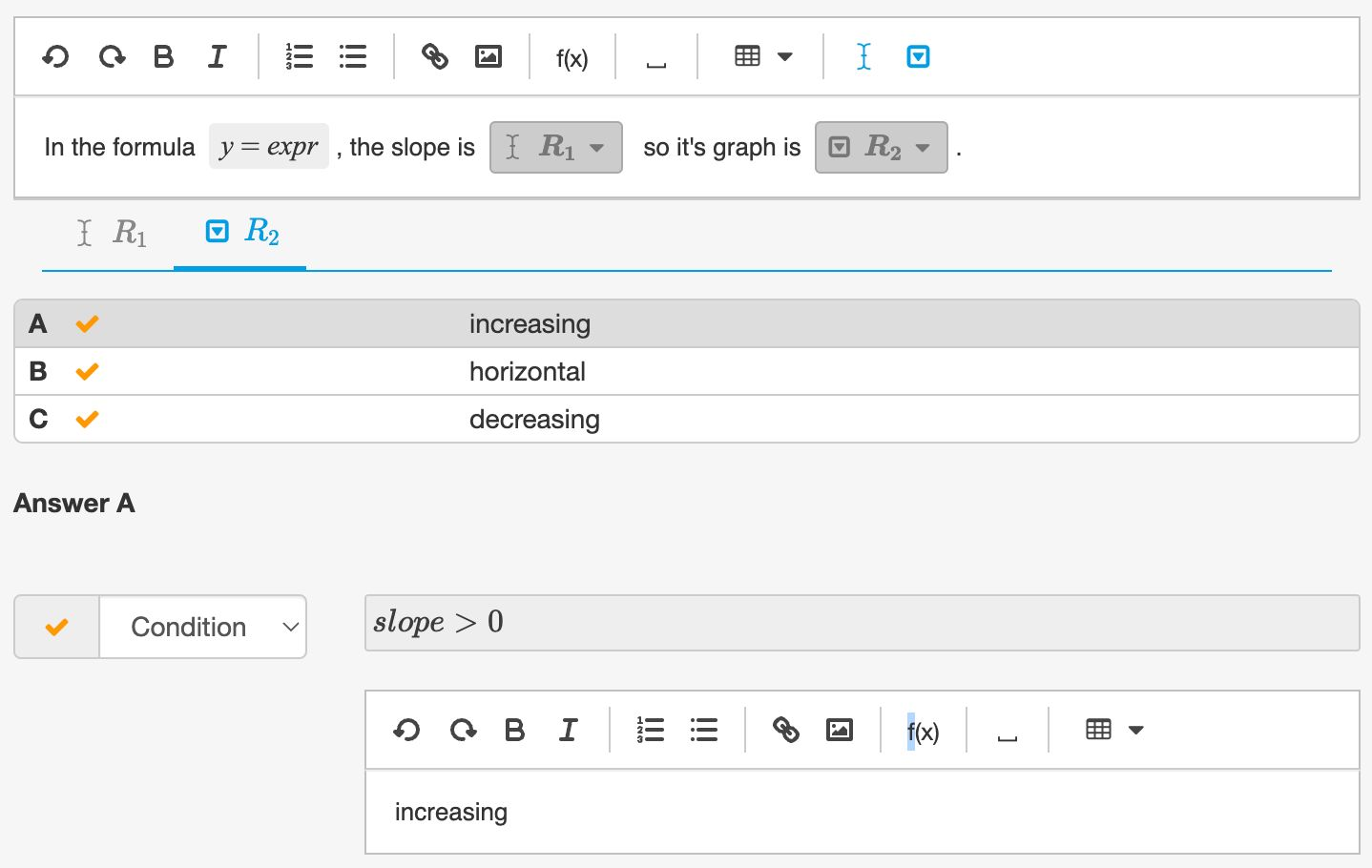# Randomizing Fill in the Blanks

## Similar approach as Multistep

You can create random instances of Fill in the Blanks questions using the same approach as for Multistep.

• Define exercise parameters in the top bar of the authoring tool
• Use these exercise parameters in the math expressions in the Fill in the Blanks question.

## An example

For example, let's create a random Fill in the Blanks question about linear formulas. Students have to identify the slope and indicate what the value means for the graph.

Example: A random question about linear formulas. Press the Repeat button to see another random instance

We will define the random linear formula by choosing a random slope, a random $y$-intercept, and a random variable.Figure: Defining random exercise parameters.

The correct response of the Selection blank depends on the random value of the slope. The graph increases if the slope is positive, decreases if the slope is negative, and is horizontal if the slope is zero. You can handle this by adding a condition to the selection options.Figure: Using exercise parameters in Fill in the Blanks.